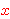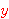Intuitionistic Logic Explorer < Previous   Next > Nearby theorems Mirrors  >  Home  >  ILE Home  >  Th. List  >  a9e Unicode version

Theorem a9e 1655
 Description: At least one individual exists. This is not a theorem of free logic, which is sound in empty domains. For such a logic, we would add this theorem as an axiom of set theory (Axiom 0 of [Kunen] p. 10). In the system consisting of ax-5 1404 through ax-14 1473 and ax-17 1487, all axioms other than ax-9 1492 are believed to be theorems of free logic, although the system without ax-9 1492 is probably not complete in free logic. (Contributed by NM, 5-Aug-1993.) (Revised by NM, 3-Feb-2015.)
Assertion
Ref Expression
a9eProof of Theorem a9e
StepHypRef Expression
1 ax-i9 1491 1Colors of variables: wff set class Syntax hints:wex 1449 This theorem was proved from axioms:  ax-i9 1491 This theorem is referenced by:  ax9o  1657  equid  1658  equs4  1684  equsal  1686  equsex  1687  equsexd  1688  spimt  1695  spimeh  1698  spimed  1699  equvini  1712  ax11v2  1772  ax11v  1779  ax11ev  1780  equs5or  1782  euequ1  2068
 Copyright terms: Public domain W3C validator